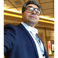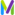cancel
Showing results for
Show  only  | Search instead for
Did you mean:

This product reached the end of support date on March 31, 2021.

What is the difference between Sum and Count with Database Execution Count measureHelper

Hi guys,

I am charting the Database Execution Count as a measure and when I toggle between Sum and Count under Aggregation I am seeing different values. The Sum values are much higher than the count values. I think the "Count" aggregate tells us how many PurePaths were calculated for this measure and "Sum" tells us the how many database executions were made in a given time frame. Is this correct or I am wrong?

Thanks,

Stan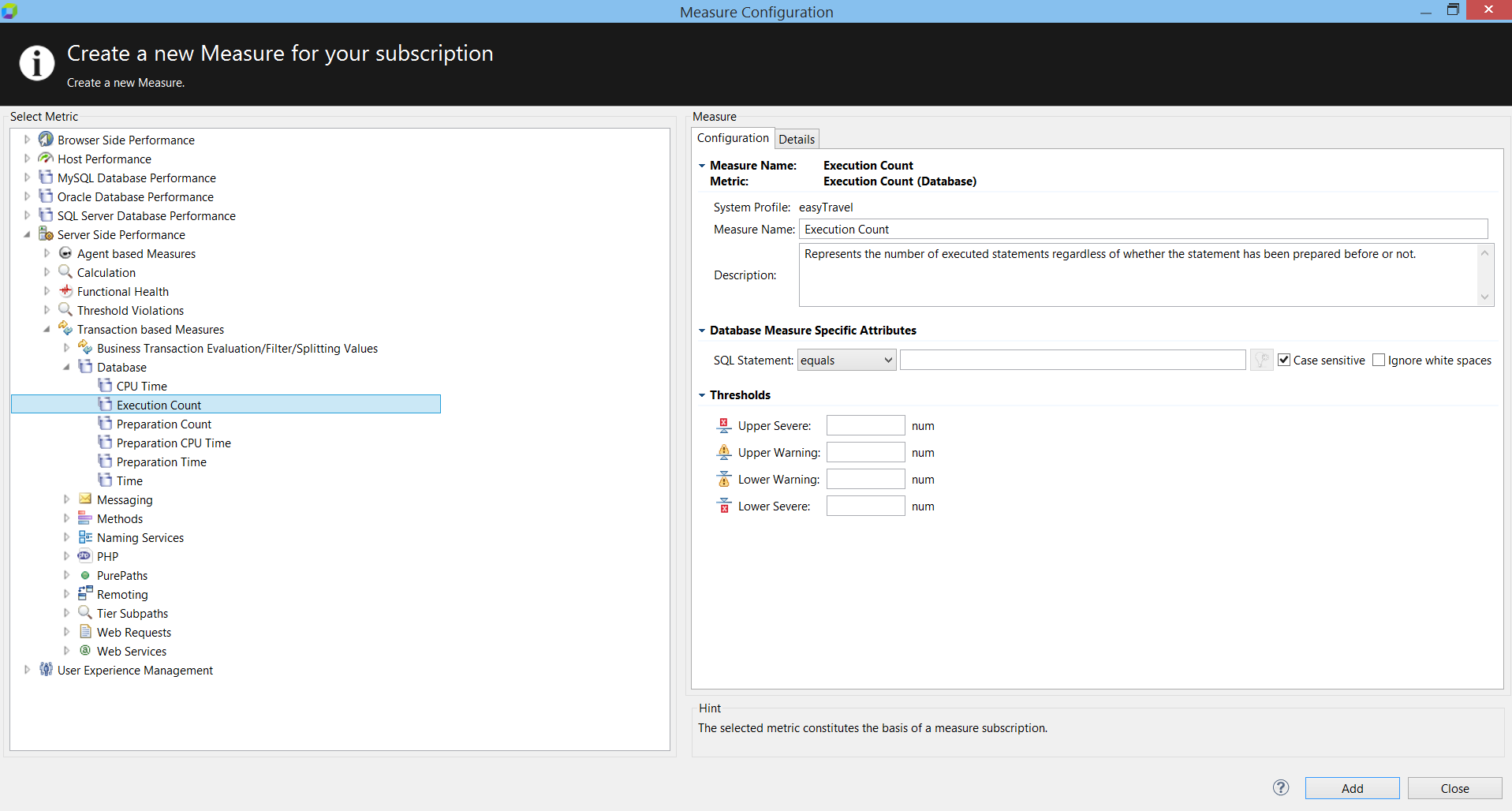6 REPLIES 6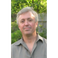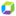Dynatrace Pro

Hi Stan,

Yes, "count" is showing you how many samples were processed in that time interval. The "sum" is, as you figured, summing up the total of the results of each sample. So if you had three of these in a 10-second interval with "execution counts" of 10, 20, and 30, the count for that interval would be 3 and the sum would be 60.

RobThanks a lot Rob.InactiveThanks Taneshaa.Participant
@Stanislav P.

Just curious, is this screenshot part of Dynatrance installed on-premise or on AWS? I was using AWS subscription, so would like to try out if this service/view is possible in AWS.